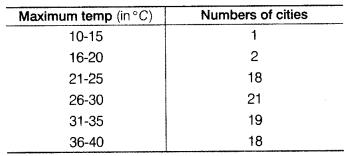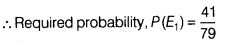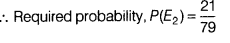# The maximum temperature is Celsius of some cities on a day are given below

The maximum temperature is Celsius of some cities on a day are given below(i)|Find the probability that maximum temperature lies between 16° Cand 30 ° C
(ii)Find the probability that minimum temperature is less than 25°C.

∵ Totalnumberofcities = 1 + 2 + 18+21+19+18 = 79
(i) Let E1 be the event, which show maximum temperature lies between 16° C and 30° C.
Number of cities = 2 + 18+21 = 41ii) Let E2 be the event, which show minimum temperature less than 25° C
Number of cities = 1 + 2 + 18 = 21